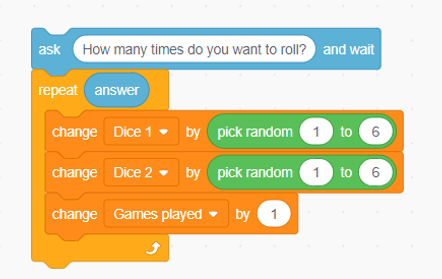top of page

# Rolling Two Dice

Lesson Overview

In this lesson, students will learn to solve problems related to probability using Scratch. They will design a game in which the program will roll two dice automatically and record the sum of all the results. During the design of the game, students have to find a way to simulate rolling two dice and be able to record the sum of all the results. The game aims to help students understand the meaning of simulation (i.e., we can use numerals to simulate rolling the two dice) and understand the mathematical concept of probability by observing the outcome of trials in the game.

### Prior Knowledge

• Mathematics: Inequality and Sum

• Scratch: Know how to use blocks such as ‘ask’, ‘forever’, ‘repeat until’, ‘if… then…’, set variables, and change size and costume.

### Learning Objectives

• To understand the role of each Sprite. In particular,

• Cat: Control the game and record the results

• Dice 1 and Dice 2: Change costumes

• To understand how to simulate rolling the two dice.

• To understand what is a good way to record the sum.

• To understand the experimental probability of getting each sum when rolling two dice.

• To understand the distribution of the sum obtained

• To understand how to check if a number is a positive integer or not (e.g., using the function ‘round’ or ‘floor’).

### Mathematics & CT Outcomes

1. Mathematics: concepts of probability, distribution of the experimental events, examination of a positive integer (e.g., using function ‘round’ or ‘mod’).

2. CT Skills: stimulation, automation, conditionals (if…then…), loops (repeat until…; forever), variable (set XX to XX; change XX by XX), operators (and; or; pick random XX to XX).

### Teaching Resources

The Desmos Activity used in this Task.

## Lesson Details

The host said that Carrie could win a jackpot if she successfully bet on the correct sum of dice when two dice were rolled. Which number should she bet on?

Let’s solve this problem using Scratch! Try to design a game to automatically roll two dice and record the sum obtained (in the area marked with '?').

Click Remix: https://scratch.mit.edu/projects/555404472.

### Guiding Questions

Upon completing the programming, students will try to understand the concept of probability by using the game to simulate the random events of rolling two dice.

1. Record the results of 5 trials with different times of rolls, e.g., 100 rolls for trial 1, 200 rolls for trial 2, etc.; students can set the number of rolls for the last trial by themselves.

2. Choose one of the above trials and plot the results in the graph. Why did you choose that trial?

3. If we roll six dice instead, describe what its corresponding distribution graph will look like in your own words. Provide at least three descriptions.

4. A game is played with the player rolling five dice and betting on the sum of dice. Which number would you bet on? Why?

#### Students' Work

There are two ways to record the sum obtained.

One is to set all the sums to different variables to record the number of occurrences; the other is to set a list to record the number of events of each sum.

##### Method 1: Setting Variables

We can take all possible outcomes of the sum of two dice (2 to 12) as independent variables and set the initial value to 0 [set XX to 0]. Then block [set Dice to pick random 1 to 6] was used to simulate the random event of dice. For recording the sum of the two dice, a custom “check result” my block is used: the result of each sum is first determined [if Dice 1+ Dice 2 = XX then XX], and then the number of occurrences of the corresponding sum is recorded [change XX by 1].##### Method 2: Setting up a List

Another way of recording and accumulating the frequency of each sum event is to set up a list with 12 rows to represent all events from sum 1 to 12. Besides, we need to use the [replace item XX of XX with XX] block to accumulate the frequency of the different sum events. As the sums are represented here by the No. of rows, the frequency of the first row (i.e., the sum of two dice is 1) is always kept at 0. This also provides an opportunity for students to gain a deeper understanding of the concept of probability.#### Possible Challenges and Misconceptions

##### (1) Difficulties in coordinating multiple sprites to perform tasks at the same timeIn this dice rolling game, students have to coordinate three sprites to handle instructions simultaneously (i.e., the cat and the two dice). They, therefore, need to set up random events for the dice rolls in the right places and change the costume in response to the number of dice in the two dice sprites. There are several ways to handle these events, such as codes in Figure 3, Figure 5, and Figure 6.

##### (2) Difficulties in simulating random events with diceIn the work, we can use [set XX to XX] and [pick random XX to XX] blocks to simulate the random event of rolling a dice. While some students may misuse the [change XX by XX] block.

##### (3) Difficulty in recording and accumulating the frequency of each sum event

As mentioned in the previous section, we can use both set variables and set lists to record the results of the rolls. However, when using the set list method, it can be a challenge for students to accumulate the frequency of each sum event.

## Acknowledgement

The author would like to thank Ka Ying LIU for designing this lesson and appreciate all the anonymous teachers and students who participated in this research.

bottom of page